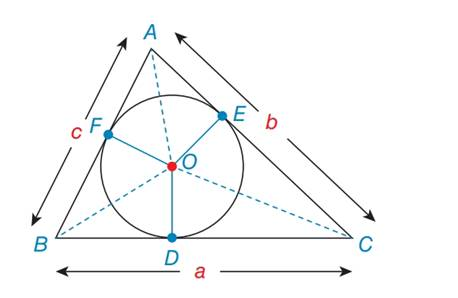Chapter 7.2, Problem 46EElementary Geometry For College St...

7th Edition
Alexander + 2 others
ISBN: 9781337614085

Solutions

Chapter
SectionElementary Geometry For College St...

7th Edition
Alexander + 2 others
ISBN: 9781337614085
Textbook Problem

For exercises 44 to 46, O is the inscribed circle for ∆ A B C . In exercises 45 and 46, use the result from 44.Where s = 1 2 a + b + c , Show that A F = s - aTo determine

To show:

That the incenter triangle AF=s-a

Explanation

Formula used:

Perimeter of incircle:

perimeterofincircle (s)=12(a+b+c)

Given:

Where s=12a+b+c, Show that AF=s-a

Calculation:

Let us consider the incircle triangle,

Let BF=x as shown in the figure.

BF=BD=x Tangents drawn from the same point B to the incircle.

Similarly, CE=CD=z and AE=AF=y

Given that s=12(a+b+c)

s=12((x+z)+(y+z)+(x+y))s=12(2x+2y+2z)s=122(x+y+z)s=x+y+zInterc

Still sussing out bartleby?

Check out a sample textbook solution.

See a sample solution

The Solution to Your Study Problems

Bartleby provides explanations to thousands of textbook problems written by our experts, many with advanced degrees!

Get Started

In Exercises 13-20, sketch a set of coordinate axes and plot each point. 15. (3, 1)

Applied Calculus for the Managerial, Life, and Social Sciences: A Brief Approach

Find the differential of each function. 12. (a) y=1+2u1+3u (b) y = 2 sin 2

Single Variable Calculus: Early Transcendentals, Volume I

Differentiate the functions in Problems 3-20. 12.

Mathematical Applications for the Management, Life, and Social Sciences

Find the value of c if n=2(1+c)n=2

Calculus (MindTap Course List)

Compute P5,2.

Understanding Basic Statistics

Find all prime ideals of .

Elements Of Modern Algebra